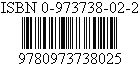A nice Website:
¤Friday the 13th¤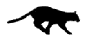HOME
 Translators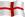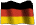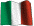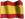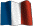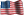Origins
 Various
 Ancients
 Modern I
 Modern II
 666: Beast
 999: Anti-Beast
 Bars Codes
 Devil's Square
 God - Trinity
 Maths 666
 References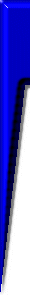χξς 666 DCLXVI וסם ﻭﺱﺥ Greek Latin Roman Hebrew Arab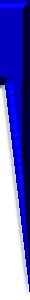Webmaster:

Devil's square 6x6

 1 2 3 4 5 6 21 = 7 x 3 7 8 9 10 11 12 57 = 19 x 3 13 14 15 16 17 18 93 = 31 x 3 19 20 21 22 23 24 129 = 43 x 3 25 26 27 28 29 30 165 = 55 x 3 31 32 33 34 35 36 201 = 67 x 3 96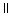16 x 6 10217 x 6 10818 x 6 11419 x 6 12020 x 6 12621 x 6 Vertical and horizontalSUMS

The square of the devil is a square 6x6 composed of the first 36 integers, whose sum is exactly equal to 666. Number 36 comprises in oneself the two basic components of triple (3) six (6). Actually, 36 is the triangular root of 666 (or secret value of 666). Let us recall here that 6 is a triangular number, because of form Tn = ½ [n(n+1)], n being a natural entirety. Triple 6 is also a triangular number.

6 = T3 = 3(3+1)/2 = (9 + 3)/2 = 12/2
666 = T36 = T6x6 = 36(36 + 1)/2 = (362 + 36)/2 = (1296 + 36)/2 = 1332/2

Also let us note that   36 = 6 x 6,  36 = 13 + 23 + 33   and  36 = 3! x 6
whereas    1 + 2 + 3 = 6 = 1 x 2 x 3
(the sequence of number 123 intervenes 3 times: 1 time in 36 and 2 times in 6! ...)

 1+2+3+4+5+6+7+8+9+10+11+12+13+14+15+16+17+18+19+20+21+22+23+24+25+26+27+28+29+30+31+32+33+34+35+36 = 666

The sums of the horizontal lines are all of the odd numbers (21, 57, 93, 129, 165, 201), whose common divider is 3; whereas the vertical sub-totals all are of the even numbers (96, 102, 108, 114, 120, 126), whose common divider is 6. These properties of the vertical and horizontal sums also determine triple six: 666, 3 times 6. We then find the figures 3 and 6 already contained in the initial number 36. In addition, the total of the vertical sums as that of the horizontal sums gives 666.

 21 + 57 + 93+ 129 + 165 + 201 = 666

 96 + 102 + 108 + 114 + 120 + 126 = 666

Figure 3 is also the numerical root of each of the horizontal sums of the diabolic square:

 21 ⇒ 2 + 1 = 3 57 ⇒ 5 + 7 = 12 ⇒ 1 + 2 = 3 93 ⇒ 9 + 3 = 12 ⇒ 1 + 2 = 3 129 ⇒ 1 + 2 + 9 = 12 ⇒ 1 + 2 = 3 165 ⇒ 1 + 6 + 5 = 12 ⇒ 1 + 2 = 3 201 ⇒ 2 + 1 = 3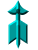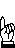In this square of the devil, the numerical roots associated with the vertical and horizontal sums of the multipliers of the respective common dividers 3 and 6 are, in the order, 6 (222 => 2 + 2 + 2 = 6) and 3 (111 => 1 + 1 + 1 = 3), that is an opposite order!... That constitutes another generating index of triple (3) six (6).

 16 + 17 + 18 + 19 + 20 + 21 = 111

 7 + 19 + 31 + 43 + 55 + 67 = 222

 111 x 6 = 666

 222 x 3 = 111 x 2 x 3 = 111 x 6 = 666

The total of the terms of the downward diagonal is identical to the sum of terms of the ascending diagonal, that is to say 111 and with numerical root equal to 3.

 1 + 8 + 15 + 22 + 29 + 36 = 111

 31 + 26 + 21 + 16 + 11 + 6 = 111

In the heart of the diabolic square (15, 16, 21, 22), the total of the terms of the downward diagonal (15,22) is identical to the sum of terms of the ascending diagonal (21,16), that is to say sum 37. The terms composing the four corners of the diabolic square (1, 6, 31, 36), added two to two also give sum 37.

 15 + 22 = 37
 21 + 16 = 37
 1 + 36 = 37
 31 + 6 = 37

The value of the numerical root associated with the cross sums of the heart of the diabolic square, the unit, relates identical to that of the cross sums of the corners of the square, because 37 => 3 + 7 = 10 => 1. This unit is contained 3 times in the diagonal-sums of the square (equal 111), while the numerical root relating to these lasts is still 3, because 111 => 1 + 1 + 1 = 3. Let us recall that the three nonunit dividers of 666 are 2, 3 and 37 (the largest). The table below gives a lighting on other intimate links between the 37, the 3, the 7 and the 666.

 666 = 18 x 37 6 + 6 + 6 = 18 666 = 6 x 3 x 37 6 + 6 + 6 = 8 + 10 666 = 2 x 3 x 3 x 37 6 + 6 + 6 = 2 + 3 + (3 + 7)

The 666 is a Number of Smith, mathematical qualifier associated with the two properties described in the last line of the table, relative to the 666 and its own dividers (2 and 3).

In addition, the first 36 integers make a magic square, qualified of "Sun Magic Square", whose sum of the terms is 666. The vertical, horizontal and diagonal sums of all its lines are each one equal to 111.

In the heart of the diabolic square (15, 16, 21, 22 like above!), the total of the terms of the downward diagonal is identical on the sum of terms of the ascending diagonal, that is to say sum 37. Terms composing the two series of four corners of the diabolic square (1, 6, 31, 36 and 8, 11, 26 29 like above!), added two to two also give sum 37. We point out that this last number, just like 36, is closely related to triple 6:     666 = 18 x 37   and   36 = 3! x 6
 1 32 34 3 35 6 30 8 27 28 11 7 20 24 15 16 13 23 19 17 21 22 18 14 10 26 12 9 29 25 31 4 2 33 5 36
 6 32 3 34 35 1 7 11 27 28 8 30 19 14 16 15 23 24 18 20 22 21 17 13 25 29 10 9 26 12 36 5 33 4 2 31

 16 + 21 = 37 22 + 15 = 37 6 + 31 = 37 36 + 1 = 37 11 + 26 = 37 29 + 8 = 37666: Bivalent Myth